Ex 2.4 (Optional)

Chapter 2 Class 10 Polynomials
Serial order wise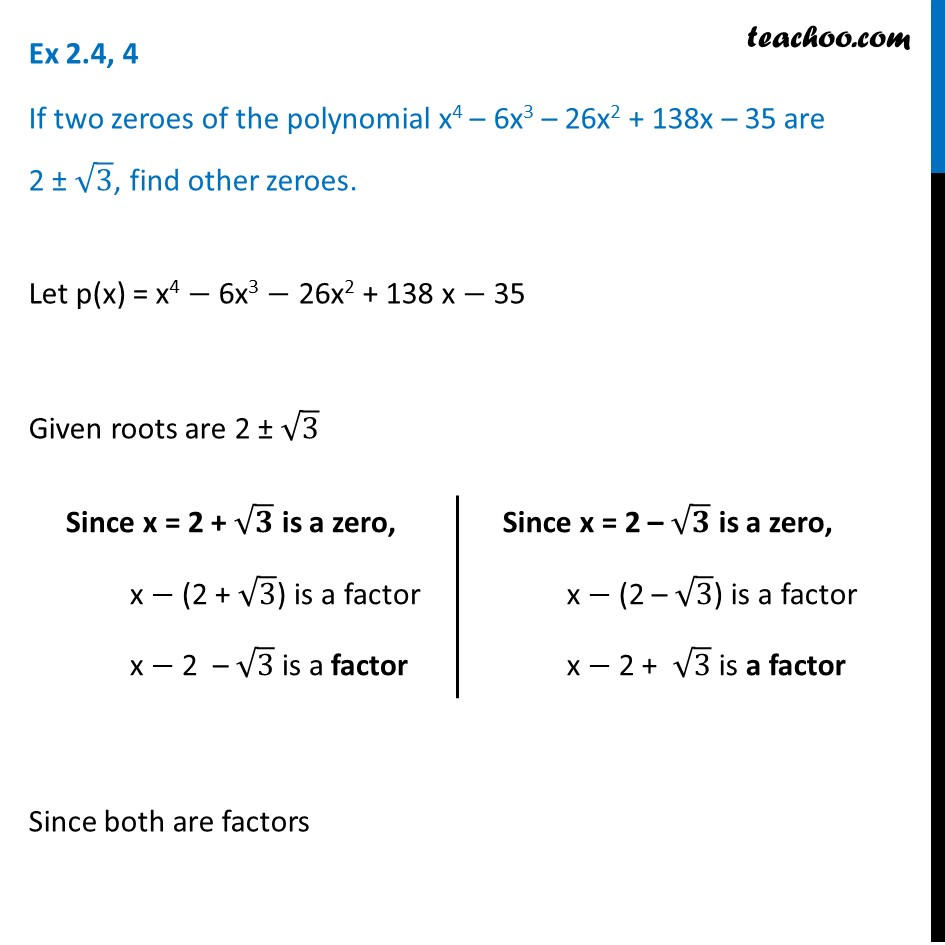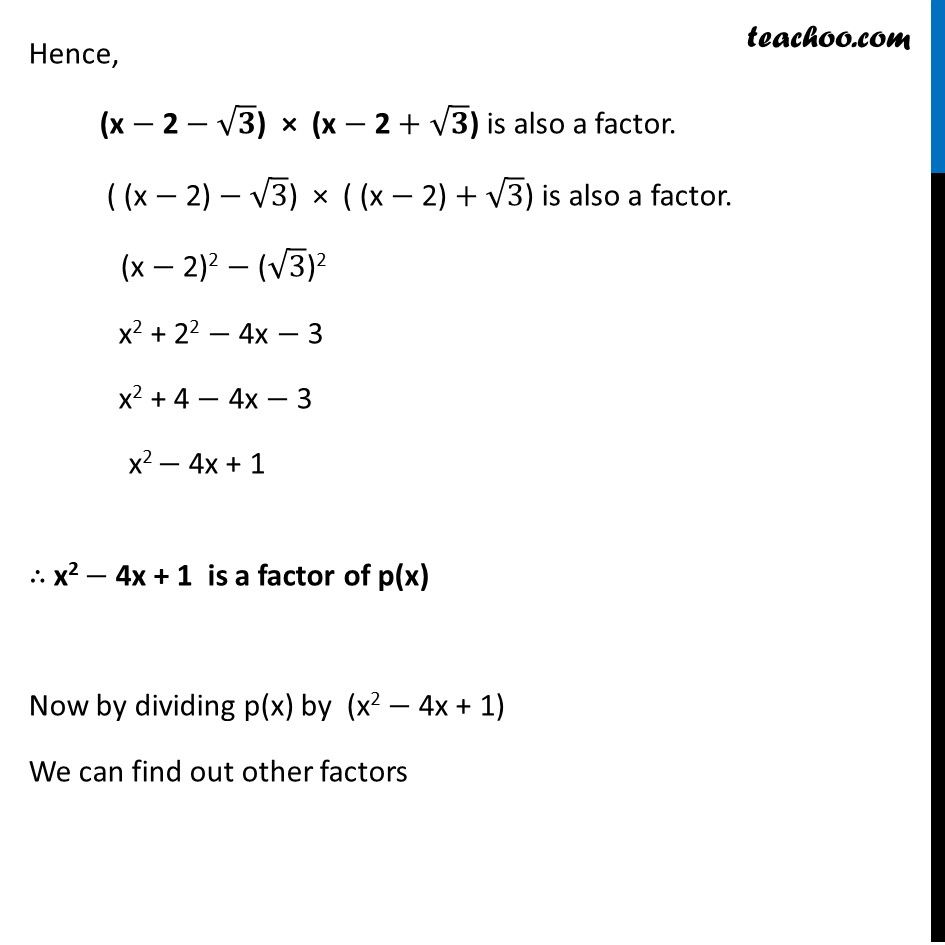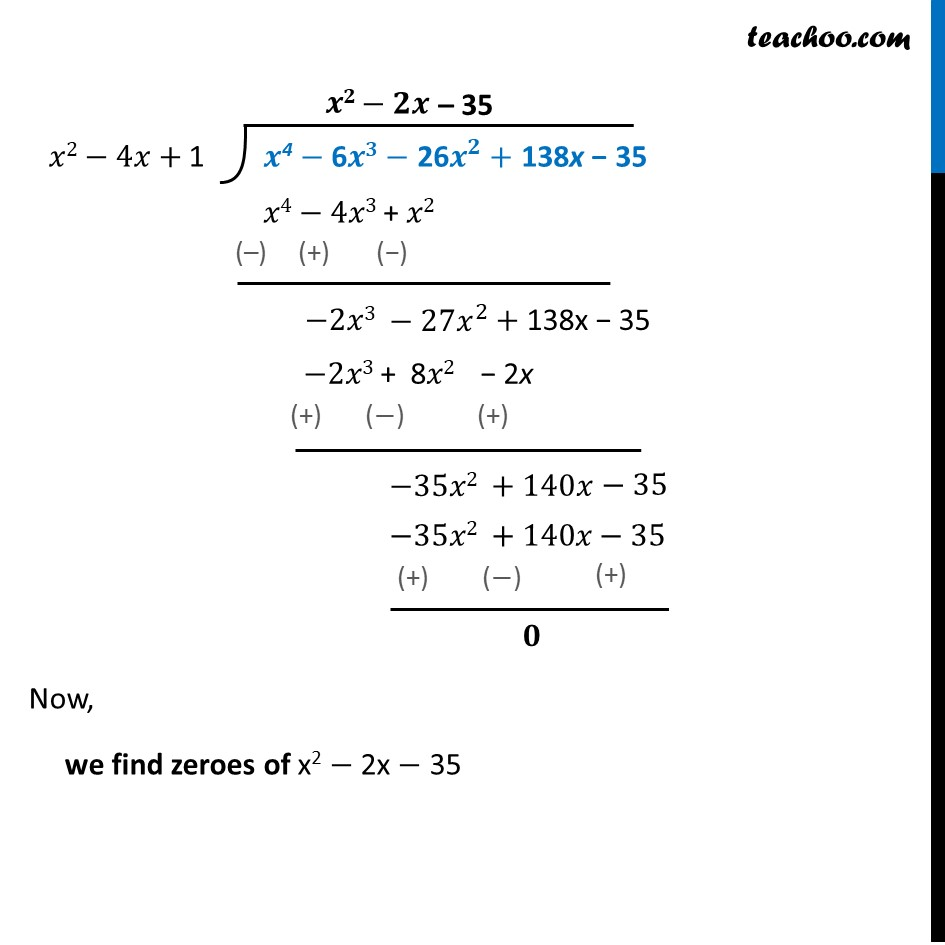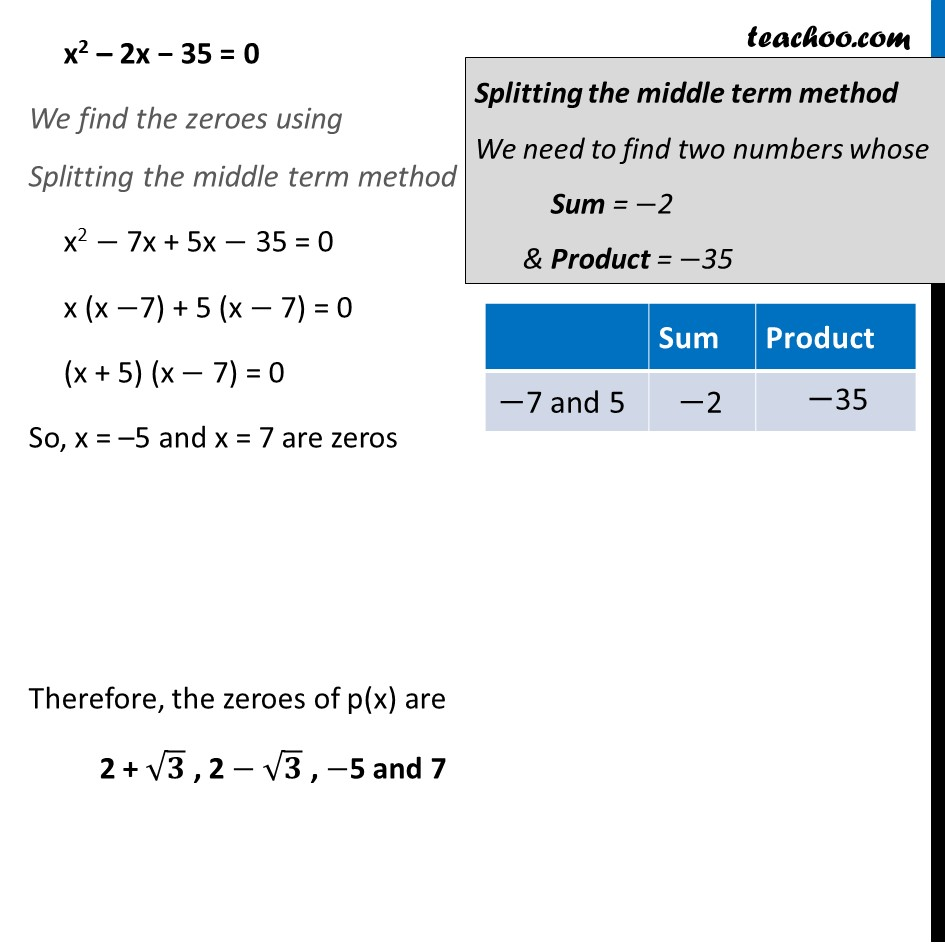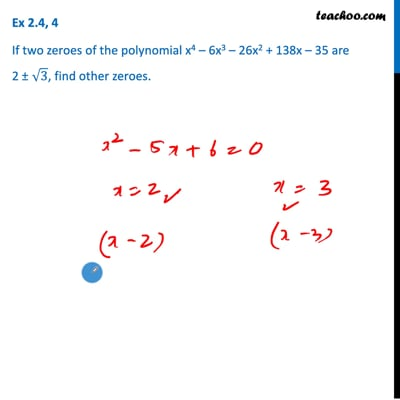This video is only available for Teachoo black users

Maths Crash Course - Live lectures + all videos + Real time Doubt solving!

### Transcript

Ex 2.4, 4 If two zeroes of the polynomial x4 – 6x3 – 26x2 + 138x – 35 are 2 ± √3, find other zeroes. Let p(x) = x4 − 6x3 − 26x2 + 138 x − 35 Given roots are 2 ± √3 Since both are factors Since x = 2 + √𝟑 is a zero, x − (2 + √3) is a factor x − 2 – √3 is a factor Since x = 2 – √𝟑 is a zero, x − (2 – √3) is a factor x − 2 + √3 is a factor Hence, (x − 2 − √𝟑) × (x − 2 + √𝟑) is also a factor. ( (x − 2) − √3) × ( (x − 2) + √3) is also a factor. (x − 2)2 − (√3)2 x2 + 22 − 4x − 3 x2 + 4 − 4x − 3 x2 − 4x + 1 ∴ x2 − 4x + 1 is a factor of p(x) Now by dividing p(x) by (x2 − 4x + 1) We can find out other factors 𝑥2−4𝑥+ 1 − 𝟐𝒙 𝒙4 − 6𝒙𝟑 − 26𝒙^𝟐+ 138x − 35 Now, we find zeroes of x2 − 2x − 35 x2 – 2x − 35 = 0 We find the zeroes using Splitting the middle term method x2 − 7x + 5x − 35 = 0 x (x −7) + 5 (x − 7) = 0 (x + 5) (x − 7) = 0 So, x = –5 and x = 7 are zeros Therefore, the zeroes of p(x) are 2 + √𝟑 , 2 − √𝟑 , −5 and 7 Splitting the middle term method We need to find two numbers whose Sum = −2 & Product = −35